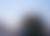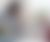# Interest Rate Roof

Our interest rate roof option offers our non-indexed customers a safety net when interest rates rise.

# Next steps

Do you want to hedge yourself against possible interest rate increases?

# How does the interest rate roof work?

• You choose the interest rate roof yourself. The amount must be over 7.5% of the annual interest rate.

• If interest rises above the roof you have requested then the interest due is altered to accommodate this. The outstanding interest (the difference between the interest charged according to the interest table and the interest rate roof you have requested) is added on to the principal amount.

• The outstanding interest is paid during the remainder of the loan period i.e. distributed payments.

• The interest rate roof is suitable for those who want to secure even payments, despite any interest rise.## Examples of effectiveness:

A.

When the interest is lower or equal to the roof, then the amount is calculated using the interest table.

Example: The interest rate rises from 6.15% to 7.40% and the roof is set at 7.5% (annual interest)

=> all interest is paid and nothing is added to the principle amount

B.

If the interest rate rises above the roof you have requested then the interest due is altered to accommodate this. The outstanding interest (the difference between the interest charged according to the interest table and the interest rate roof you have requested) is added on to the principal amount.
Example: The interest rate rises to 8.5% and the set roof is 7.5% (annual interest)

=> The paid interest is 7.5% and the remaining 1% is added on to the principal amount.

Compare the pros and cons of the interest rate roof.

 Pros Cons Reduces uncertainty and offers security. If the interest rises above the interest rate roof then the interest accumulates and is paid later. An easier option for those who choose the non-indexed loan route, despite higher interest rates. The principal amount will rise beyond initial expectations if the interest rate is higher than the roof. Quicker asset growth when compared with indexed mortgages- if the interest rate develops in the same way as the rate of inflation. The total cost of the mortgage rises when the principal amount rises. The customer decides the interest rate roof and can adjust payments according to payment ability at any given time. The long-term debt-burden increases when outstanding interest is added to the principal amount, but payments are distributed across the entire outstanding loan period.

The minimum interest rate roof is specified in the terms of the mortgage (or registered appendix) and is valid for 10 years after it has been signed.